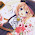## 2017年4月27日 星期四

### 等速圓周運動

#### 5 則留言:

1.設繞行圓心O，∠ACO=φ，則所求θ=π/2 -φ
繞行半徑r，角速率ω，向心加速度a
a=gtanφ=ω^2r
⇒ω=sqrt(gtanφ/r)=sqrt(gtanφ/Lsinφ)=sqrt(g/Lcosφ)

v=ωr
⇒v=sqrt(g/Lcosφ)‧Lsinφ=sqrt(gLsin^2φ/cosφ)
⇒v^2=gLsin^2φ/cosφ
⇒v^2cosφ=gL(1-cos^2φ)=gL-gLcos^2φ
⇒-gLcos^2φ-v^2cosφ+gL=0
⇒gLcos^2φ+v^2cosφ-Lg=0
代公式解
cosφ=-v^2±sqrt(v^4+4(gL)^2) /2gL
φ為銳角，取正解。
⇒φ=arccos[-v^2±sqrt(v^4+4(gL)^2) /2gL]

⇒θ=π/2 -φ=π/2-arccos[-v^2±sqrt(v^4+4(gL)^2) /2gL]

對了，市賽科展獲得了佳作與研究精神獎。

1.上面是我的留言，只是不知道為什麼變成那樣。

2.留言變 Unknown，我也不清楚，通常是選擇匿名發表。

很棒的解法，我是直接用θ，沒有φ，然後解方程式 gLsin^2θ+v^2sinθ-Lg=0，你試看看

恭喜市科展獲獎，請問市科展比賽仍是數學、理化、生活科學等混合比賽嗎?

3.現在是分科比賽了，也是分科敘獎。

4.能得獎就很厲害